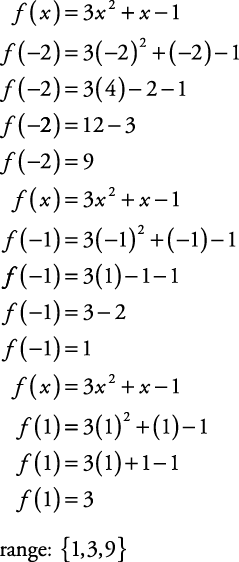## Functions

Functions are very specific types of relations. Before defining a function, it is important to define a relation.

#### Relations

Any set of ordered pairs is called a relation. Figure 1 shows a set of ordered pairs.

A = {(–1, 1), (1, 3), (2, 2), (3, 4)}

Figure 1. A graph of the set of ordered pairs (–1, 1), (1, 3), (2, 2), (3, 4).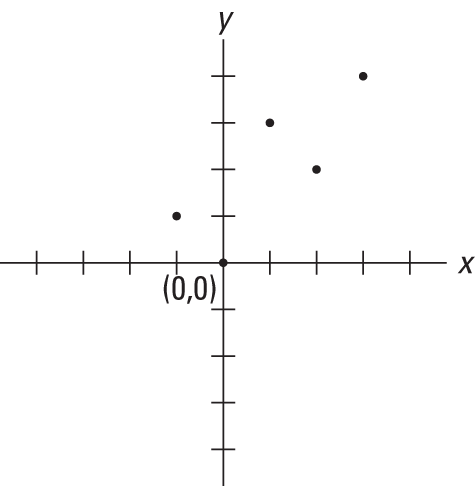#### Domain and range

The set of all x's is called the domain of the relation. The set of all y's is called the range of the relation. The domain of set A in Figure 1 is {–1, 1, 2, 3}, while the range of set A is {1, 2, 3, 4}.

##### Example 1

Find the domain and range of the set of graphed points in Figure 2.

The domain is the set {–2, –1, 1, 3}. The range is the set {–1, 2, 3}.

Figure 2. Plotted points.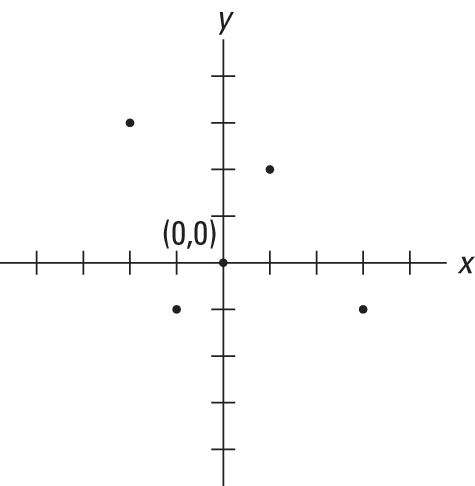#### Defining a function

The relation in Example has pairs of coordinates with unique first terms. When the x value of each pair of coordinates is different, the relation is called a function. A function is a relation in which each member of the domain is paired with exactly one element of the range. All functions are relations, but not all relations are functions. A good example of a functional relation can be seen in the linear equation y = x + 1, graphed in Figure 3. The domain and range of this function are both the set of real numbers, and the relation is a function because for any value of x there is a unique value of y.

Figure 3. A graph of the linear equation y = x + 1.#### Graphs of functions

In each case in Figure 4 (a), (b), and (c), for any value of x, there is only one value for y. Contrast this with the graphs in Figure 5.

Figure 4. Graphs of functions.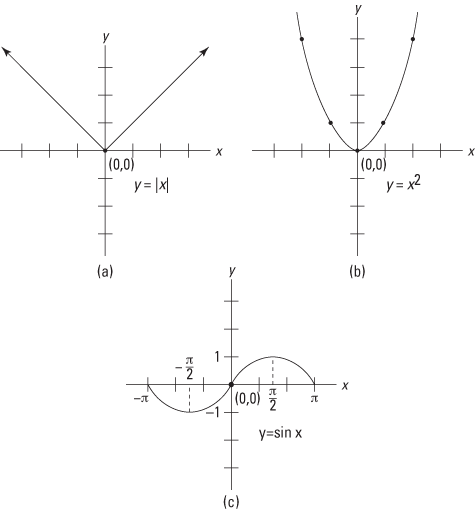#### Graphs of relationships that are not functions

In each of the relations in Figure 5 (a), (b), and (c), a single value of x is associated with two or more values of y. These relations are not functions.

Figure 5. Graphs of relations that are not functions.#### Determining domain, range, and if the relation is a function

##### Example 2

1.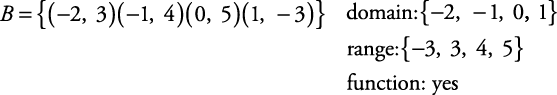2.3.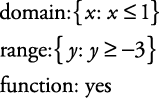4.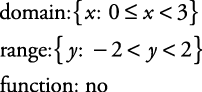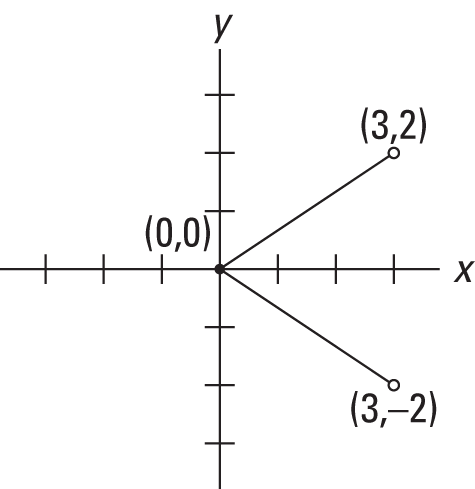5.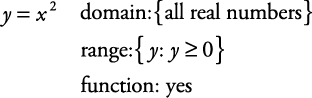6.Note that Examples 2(e) and (f) are illustrations of inverse relations: relations in which the domain and the range have been interchanged. Notice that while the relation in (e) is a function, the inverse relation in (f) is not.

#### Finding the values of functions

The value of a function is really the value of the range of the relation. Given the function

f = {(1, –3), (2, 4), (–1, 5), (3, –2)}

the value of the function at 1 is –3, at 2 is 4, and so forth. This is written f(1) = –3 and f(2) = 4 and is usually read, “ f of 1 = –3 and f of 2 = 4.” The lowercase letter f has been used here to indicate the concept of function, but any lowercase letter might have been used.

##### Example 3

Let h = {(3, 1), (2, 2), (1, –2), (–2, 3)} Find each of the following.

1.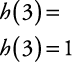2.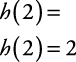3.4.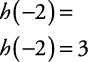##### Example 4

If g( x) = 2 x + 1, find each of the following.

1.2.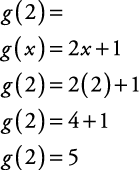3.##### Example 5

If f ( x) = 3 x 2 + x –1, find the range of f for the domain {–2, –1, 1}.